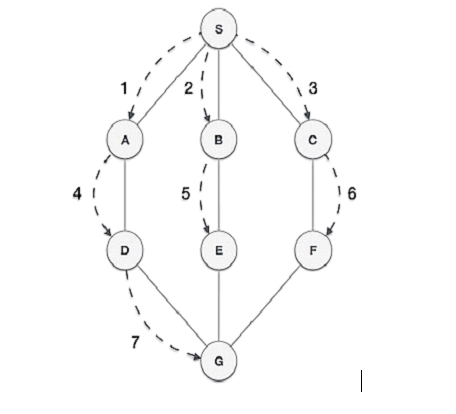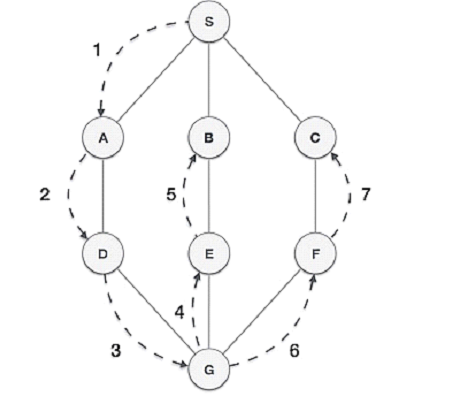# Difference between BFS and DFS

Both BFS and DFS are types of graph traversal algorithms, but they are different from each other. BFS or Breadth First Search starts from the top node in the graph and travels down until it reaches the root node. On the other hand, DFS or Depth First Search starts from the top node and follows a path to reaches the end node of the path.

## What is BFS?

Breadth First Search (BFS) algorithm traverses a graph in a breadth-ward motion and uses a queue to remember to get the next vertex to start a search when a dead end occurs in any iteration.

BFS is basically a nodebased algorithm which is used to find the shortest path in the graph between two nodes. BFS moves through all of its nodes which are connected to the individual nodes.

BFS uses the FIFO (First In First Out) principle while using the Queue to find the shortest path. However, BFS is slower and requires a large memory space.

### Example of BFS## What is DFS?

Depth First Search (DFS) algorithm traverses a graph in a depth-ward motion and uses a stack to remember to get the next vertex to start a search when a deadend occurs in any iteration.

DFS uses LIFO (Last In First Out) principle while using Stack to find the shortest path. DFS is also called Edge Based Traversal because it explores the nodes along the edge or path. DFS is faster and requires less memory. DFS is best suited for decision trees.

### Example of DFS## Difference between BFS and DFS

The following are the important differences between BFS and DFS −

Key

BFS

DFS

Definition

BFS stands for Breadth First Search.

DFS stands for Depth First Search.

Data structure

BFS uses a Queue to find the shortest path.

DFS uses a Stack to find the shortest path.

Source

BFS is better when target is closer to Source.

DFS is better when target is far from source.

Suitability for decision tree

As BFS considers all neighbor so it is not suitable for decision tree used in puzzle games.

DFS is more suitable for decision tree. As with one decision, we need to traverse further to augment the decision. If we reach the conclusion, we won.

Speed

BFS is slower than DFS.

DFS is faster than BFS.

Time Complexity

Time Complexity of BFS = O(V+E) where V is vertices and E is edges.

Time Complexity of DFS is also O(V+E) where V is vertices and E is edges.

Memory

BFS requires more memory space.

DFS requires less memory space.

Tapping in loops

In BFS, there is no problem of trapping into finite loops.

In DFS, we may be trapped into infinite loops.

Principle

BFS is implemented using FIFO (First In First Out) principle.

DFS is implemented using LIFO (Last In First Out) principle.

## Conclusion

Both BFS and DFS are graph traversal algorithms. The most significant difference between the two is that the BFS algorithm uses a Queue to find the shortest path, while the DFS algorithm uses a Stack to find the shortest path.